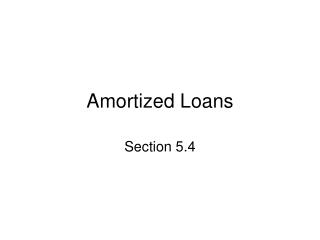DownloadDownload PresentationAmortized Loans

# Amortized Loans

Télécharger la présentation## Amortized Loans

- - - - - - - - - - - - - - - - - - - - - - - - - - - E N D - - - - - - - - - - - - - - - - - - - - - - - - - - -
##### Presentation Transcript

1. Amortized Loans Section 5.4

2. Introduction • The word amortize comes from the Latin word admoritz which means “bring to death”. • What we are saying is that we want to bring the debt to death! More gently it is retiring the debt. • The important factors related to an amortized loan are the principal, annual interest rate, the length of the loan and the monthly payment. • If we know any 3 of the above factors, the fourth can be found.

3. Charting the history of a loan • Chart the history of an amortized loan of \$1000 for three months at 12% interest, with a monthly payment of \$340. • When the 1st payment is made 1/12 of a year has gone by, so the interest is \$1000 x .12 x 1/12 = \$10. • The payment first goes toward paying the interest, then the rest is applied to the unpaid balance. The net payment is \$340 - \$10 = \$330. • The new balance is \$1000 - \$330 = \$670. • Now we calculate the interest on the remaining balance. • \$670 x .12 x 1/12 = \$6.70. • The net payment is \$340 - \$6.70 = \$333.30. • The new balance is \$670 - \$333.30 = \$336.70. • Once again we calculate the interest on the remaining balance. • \$336.70 x .12 x 1/12 = \$3.37. • Thus the last payment has to cover the interest and the remaining balance. • This is \$3.37 + \$336.70 = \$340.07. Thus the last payment is \$340.07

4. A table of the previous example • Beginning balance \$1000

5. Finding a monthly payment • Many times we know the length of a loan, the annual interest rate and the amount of the loan. Can we afford to make the monthly payment??? This question is very important when considering a mortgage. • The monthly payment formula is basically derived from the equation future value of annuity = future value of loan amount.

6. Payment formula • Let P be present value or full amount of loan, r is the annual interest rate, t is the length of the loan and PMT is the monthly payment.

7. Example • What is the monthly payment for a loan of \$29,000 for 5 years at an annual interest rate of 5%. • The monthly payment is \$547.27 • Note: If you follow this schedule, you will make 60 payments of \$547.27 which in total is \$32836.20. The amount of interest paid to the lender is \$32836.20 - \$29000 = \$3836.20

8. Example using Table 1 • Amortization tables have been created so that people don’t need to use the complicated payment formula. • For example, find the monthly payment for a \$10000 loan at 10% annual interest for 5 years. • Looking at Table 1, this corresponds to the entry of \$212.48. • Verify using the PMT formula. You may be off by a cent or two, that’s because rounding error was introduced into the table.

9. Another example using table 1 • What would be the payment on a loan of \$58,000 at 10% annual interest for 30 years? • \$58000 = \$50000 + 4 x \$2000 • We will use the entries for \$50000 at 30 years and \$2000 at 30 years. • The PMT = \$438.79 + 4 x \$17.56 = \$509.03 • Verify using the PMT formula. Rounding error has been introduced.

10. Table 1 - Amortization Table at 10%

11. Example Using Table 2 • Recall that we calculated the monthly payment of a \$29000 loan for 5 years at 5% annual interest to be \$547.27. • Let’s use table 2. • The entry that corresponds to 5% for 5 years is \$18.871234. • Since this is a \$1000 table, and the loan amount is for \$29000, we multiply the \$18.871234 by 29 to get a monthly payment of \$547.265786 or properly \$547.27. The same as we computed using the formula.

12. Table 2 - Amortization Table for \$1000 Loan